Courses

# Test: Average, Ratio & Proportion

## 20 Questions MCQ Test Topic-wise Past Year Questions for CAT | Test: Average, Ratio & Proportion

Description
This mock test of Test: Average, Ratio & Proportion for CAT helps you for every CAT entrance exam. This contains 20 Multiple Choice Questions for CAT Test: Average, Ratio & Proportion (mcq) to study with solutions a complete question bank. The solved questions answers in this Test: Average, Ratio & Proportion quiz give you a good mix of easy questions and tough questions. CAT students definitely take this Test: Average, Ratio & Proportion exercise for a better result in the exam. You can find other Test: Average, Ratio & Proportion extra questions, long questions & short questions for CAT on EduRev as well by searching above.
QUESTION: 1

### The salaries of Ramesh, Ganesh and Rajesh were in the ratio 6:5:7 in 2010, and in the ratio 3:4:3 in 2015. If Ramesh’s salary increased by 25% during 2010-2015, then the percentage increase in Rajesh’s salary during this period is closest to (2019)

Solution:

Let salaries of Ramesh, Ganesh and Rajesh in 2010 be 6x, 5x and 7x respectively.
Also, let salaries of Ramesh, Ganesh and Rajesh in 2015 be 3y, 4y and 3y respectively
Given, 3y = 1.25 × 6x ⇒ = 2.5x
∴ Salary of Rajesh in 2015 = 3y = 3 × 2.5x = 7.5x
∴ Percentage increase in salary of Rajesh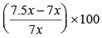≈ 7

QUESTION: 2

### The average of 30 integers is 5. Among these 30 integers, there are exactly 20 which do not exceed 5. What is the highest possible value of the average of these 20 integers? (2019)

Solution:

Let a be  the average of 20 numbers whose average does not exceed 5.
Let b be  the average of rest of the 10 numbers. Clearly,
b > 5 i.e. the average of these numbers exceeds 5
Therefore,
30 × 5 = 20a + 10b
⇒ 2a + b = 15
⇒ b = 15 – 2a
Going by the options, we can say that when a = 4.5, then b = 6 which satisfies all the conditions.
But when a = 5, then b = 5 which does not satisfies b > 5
Hence, highest possible value of the required average = 4.5

QUESTION: 3

### In an examination, Rama’s score was one-twelfth of the sum of the scores of Mohan and Anjali. After a review, the score of each of them increased by 6. The revised scores of Anjali, Mohan, and Rama were in the ratio 11 : 10 : 3. Then Anjali’s score exceeded Rama’s score by (2019)

Solution:

Since scores of Anjali, Mohan and Rama after review were in the ratio of 11 : 10 : 3, therefore we can suppose scores of Anjali, Mohan and Rama after review be 11x, 10x and 3x respectively.
Therefore, their scores before review was (11x – 6), (10x – 6) and (3x – 6) respectively.
Since, Rama’s score was one-twelfth of the sum of the scores of Mohan and Anjali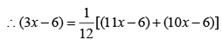⇒ 12 (3x – 6) = 21x – 12 ⇒ x = 4
Now, Anjali’s score – Rama’s score
= (11x – 6) – (3x – 6) = 8x = 8 × 4 = 32

QUESTION: 4

The strength of a salt solution is p% if 100 ml of the solution contains p grams of salt. Each of three vessels A, B, C contains 500 ml of salt solution of strengths 10%, 22%, and 32%, respectively. Now, 100 ml of the solution in vessel A is transferred to vessel B. Then, 100 ml of the solution in vessel B is transferred to vessel C. Finally, 100 ml of the solution in vessel C is transferred to vessel A. The strength, in percentage, of the resulting solution in vessel A is

(2019)

Solution:

Initial amount of salt in vessel A = 10 gms per 100 ml. solution. Therefore in 500 ml solution in vessel amount of salt = 50 gms
Similarly, initially in 500 ml solution in vessel B amount of salt = 110 gms
and initially in 500 ml solution in vessel C, amount of salt = 160 gms
When 100 ml is transferred from A to B, the amount of salt now in B = 10 + 110 = 120 gms in 600 ml.
The new concentration of salt in B = 120 / 600 x 100
= 20 gms per 100 ml.
Now, the amount of salt in A = 50 – 10 = 40 gms in 400 ml
Now, when 100 ml is transfered from B to C, the amount of salt now in C = 20 + 160 = 180 gms in 600 ml.
The new concentration of salt C = 180 / 600 x 100
= 30 gms per 100 ml
Finally, when 100 ml is transfered from C to A, the amount of salt now in A = 30 + 40 = 70 gms in 500 ml.
∴ Strength of salt in 70 / 500 x 100 = 14

QUESTION: 5

A chemist mixes two liquids 1 and 2. One litre of liquid 1 weighs 1 kg and one litre of liquid 2 weighs 800 gm. If half litre of the mixture weighs 480 gm, then the percentage of liquid 1 in the mixture, in terms of volume, is

(2019)

Solution:

Weight of liquid 1 per litre = 1000 gm
Weight of liquid 2 per litre = 800 gm
Weight of mixture per litre = 2 × 480 = 960 gm
By alligation rule,
Quantity of liquid 1 / Quantity of liquid 2 =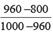= 4 / 1
Hence, the liquids are mixed in 4 : 1.
∴ Percentage of liquid 1 = (4 / 4 + 1) x 100 = 80%

QUESTION: 6

Amala, Bina, and Gouri invest money in the ratio 3 : 4 : 5 in fixed deposits having respective annual interest rates in the ratio 6 : 5 : 4. What is their total interest income (in Rs) after a year, if Bina’s interest income exceeds Amala’s by ₹ 250?

(2019)

Solution:

Ratio of incomes of Amala, Bina and Gouri = 3 : 4 : 5
Ratio of interests of Amala, Bina and Gouri = 6 : 5 : 4
Therefore, the ratio of their interest income = (3 × 6) : (4 × 5) : (5 × 4) = 18 : 20 : 20
Let the interest incomes of Amala, Bina and Gouri be 18x, 20x and 20x respectively.
Since, Bina’s interest income exceeds Amala’s by Rs 250
Therefore, 20x – 18x = 250 ⇒ x = 125
Total interest incomes = 18x + 20x + 20x = 58x = 58 × 125 = 7250

QUESTION: 7

Ramesh and Gautam are among 22 students who write an examination. Ramesh scores 82.5. The average score of the 21 students other than Gautam is 62. The average score of all the 22 students is one more than the average score of the 21 students other than Ramesh. The score of Gautam is

(2019)

Solution:

Let x be the average of all 22 students and Gautam’s score be G.
Hence, total scores of all 22 students = 22x.
From the first given condition,
22 x – G = 21 × 62 ...(i)
From the second given condition,
22x – 82.5 = 21(x – 1)
⇒ 22x – 21x = 82.5 – 21
⇒ x = 61.5
On putting x = 61.5 in (i), we get
22 × 6 1.5 – G = 21 × 62
⇒ G = 1353 – 1302 = 51

QUESTION: 8

Corners are cut off from an equilateral triangle T to produce a regular hexagon H. Then, the ratio of the area of H to the area of T is

(2019)

Solution:

Refer to the below figure.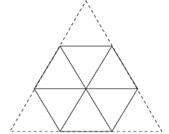As we can see, the triangle can be divided into 9 conguruent smaller equilateral triangles. Also, hexagon contains 6 of them.
∴ The required ratio = 6 : 9 = 2 : 3

QUESTION: 9

A CAT aspirant appears for a certain number of tests. His average score increases by 1 if the first 10 tests are not considered, and decreases by 1 if the last 10 tests are not considered. If his average scores for the first 10 and the last 10 tests are 20 and 30, respectively, then the total number of tests taken by him is

(2018)

Solution:

Let the average score of the aspirant in all the tests be A. Let the number of tests be N.
The aspirant’s average score for the first 10 tests and last 10 tests are 20 and 30 respectively.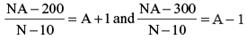⇒    10A – N = 190 and N + 10A = 310
On subtracting,
we get,  – 2N = – 120
⇒ N = 60.

QUESTION: 10

In an apartment complex, the number of people aged 51 years and above is 30 and there are at most 39 people whose ages are below 51 years. The average age of all the people in the apartment complex is 38 years. What is the largest possible average age, in years, of the people whose ages are below 51 years?

(2018)

Solution:

Let the average age of people aged 51 years and above be A1 years and the average age of people aged below 51 years be A2 years. Let the number of people aged below 51 years be N2.
The average age of all the people in the apartment complex is 38 years.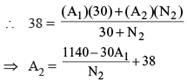Clearly for A2 to be maximum, A1 should be minimum i.e. 51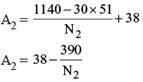Clearly for A2 to be maximum, N2 should be also maximum i.e. 39 Hence maximum value of A2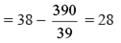QUESTION: 11

Two types of tea, A and B, are mixed and then sold at Rs. 40 per kg. The profit is 10% if A and B are mixed in the ratio 3 : 2, and 5% if this ratio is 2 : 3. The cost prices, per kg, of A and B are in the ratio.

(2018)

Solution:

Let the cost prices of A and B be Ca and Cb respectively.
Selling price of the mixture = 40 per kg.
The profit made is 10% if A and B are mixed in the ratio 3:2.
∴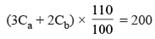⇒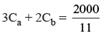The profit made is 5% if A and B are mixed in the ratio 2 : 3.
∴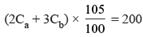⇒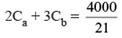Divide equation (i) by (ii), we get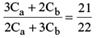⇒ 24Ca = 19Cb ⇒ Ca : Cb = 19 : 24

QUESTION: 12

Raju and Lalitha originally had marbles in the ratio 4:9. Then Lalitha gave some of her marbles to Raju. As a result, the ratio of the number of marbles with Raju to that with Lalitha became 5:6. What fraction of her original number of marbles was given by Lalitha to Raju?

(2018)

Solution:

Let the numbers of marbles with Raju and Lalitha be 4x and 9x respectively.
Let Lalitha gave y marbles to Raju.
∴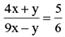⇒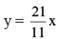Fraction of original marbles that Lalitha gave to Raju
= y / 9x = 7 / 33

QUESTION: 13

A class consists of 20 boys and 30 girls. In the mid-semester examination, the average score of the girls was 5 higher than that of the boys. In the final exam, however, the average score of the girls dropped by 3 while the average score of the entire class increased by 2. The increase in the average score of the boys is

(2017)

Solution:

Let the average score of the boys in the midsemester examination be b
Average score of the girls = b + s
in the exam,
Average score of the girl = b + 5 – 3
= b + 2
Average score of the entire class increased by 2 and is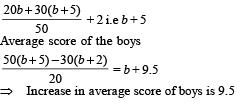QUESTION: 14

A stall sells popcorn and chips in packets of three sizes : large, super, and jumbo. The numbers of large, super, and jumbo packets in its stock are in the ratio 7 : 17 : 16 for popcorn and 6 : 15 : 14 for chips. If the total number of popcorn packets in its stock is the same as that of chips packets, then the numbers of jumbo popcorn packets and jumbo chips packets are in the ratio

(2017)

Solution:

Let the total no. of popcorn pockets in stock be T
Total no. of chips pockets in stock = T
Required ratio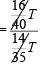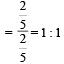QUESTION: 15

Suppose, C1, C2, C3, C4 and C5 are five companies. The profits made by C1, C2 and C3 are in the ratio 9 : 10 : 8 while the profits made by C2, C4, and C5 are in the ratio 18 : 19 : 20. If C5 has made a profit of ₹19 crore more than C1, then the total profit (in ₹) made by all five companies is

(2017)

Solution: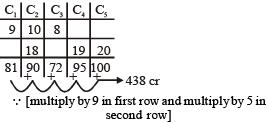C5 – C1 = 19, the numbers above are the actual profits
∴ The total profit = 438 crore.

QUESTION: 16

A certain sum of money is made up of Re. 1, 50 paise and 25 paise coins. The ratio of the number of these coins is 5 : 6 : 8. Then, 3/5th of the Re. 1 coins are changed to 50 paise and 25 paise coins, such that the ratio of the total number of these coins in the same order became 1 : 2. Now, half of the 50 paise coins are changed to Re. 1 coins and all the 25 paise coins are changed to Re.1 and 50 paise coins in the ratio 7 : 4. What is the ratio of the Re. 1 and 50 paise coins at the end of the conversions?
(Note: If you change a Re.1 coin into 50 paise coins, then you will get two coins of 50 paise for a Re. 1 coin.)

(2015)

Solution:

Let the number of Re. 1,50 paise and 25 paise coins be 360, 432 and 576 respectively (ratio 5 : 6 : 8).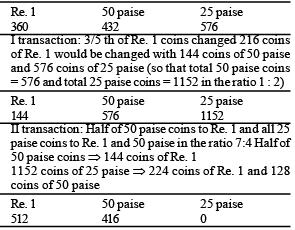Ratio = 512 : 416 =16 : 13.

QUESTION: 17

There are 40 students in a class. A student is allowed to shake hand only once with a student who is taller than him or equal in height to him. He can’t shake hand with anyone who is shorter than him. Average height of the class is 5 feet. What is the difference between the maximum and minimum number of handshakes that can take place in the class?

(2015)

Solution:

If all are of equal height. number of handshakes = 40C2.
If all are of different heights, number of handshakes = 0
∴ Required difference = 40C2 – 0 = 40C2.

QUESTION: 18

The ratio of alcohol to water in an alcohol-water solution is 9 : 1. The rate of evaporation per hour of alcohol and water on boiling is 20% and 5% respectively. The minimum number of hours for which the solution needs to be boiled so as it contains at least 18% of water?

(2013)

Solution:

Let the required number of hour be h.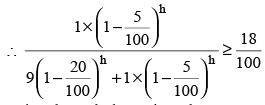Going through the options, h = 4 comes out to be the correct answer.

QUESTION: 19

Three men are gambling in Casino Royal. They start with sums of money in the ratio 7 : 6 : 5 and finish with sums of money in the ratio 6 : 5 : 4, in the same order as before. One of them won \$ 12. How many dollars did he start with ? [The three men gambled amongst each other only]

(2012)

Solution:

Let the total amount involved in this game in \$K.
The first person has (7/18)k in the beginning and (6/15)k in the end. Thus he won something.
Second person has (6/18)k in the beginning and (5/15)k in the end. So he neither gains nor loses. At this point it is very clear that third person loses something.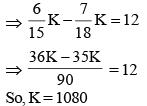So, the winner must have started. with \$ 420.

QUESTION: 20

The letters of the English alphabet, in the order A to Z, are made to represent 26 numbers which are in Arithmetic Progression. The sum of the numbers representing A, C and E is 36 while that of A, C, E and G is 60. What is the sum of the numbers representing B, D, F and H?

(2011)

Solution:

Let the number representing A be a, and the common difference be d.
⇒ a + (a + 2d) + (a + 4d) = 36 ...(i)
and (A + C + E + G) – (A + C + E) = 60 – 36
⇒ G = 24
⇒ a + 6d = 24 ...(ii)
From (i) and (ii)
(3a + 6d) – (a + 6d) = 12
⇒ 2a = 12 ⇒ a = 6 and d = 3
So, B + D + F + H = 4a + 16d
= 24 + 48 = 72